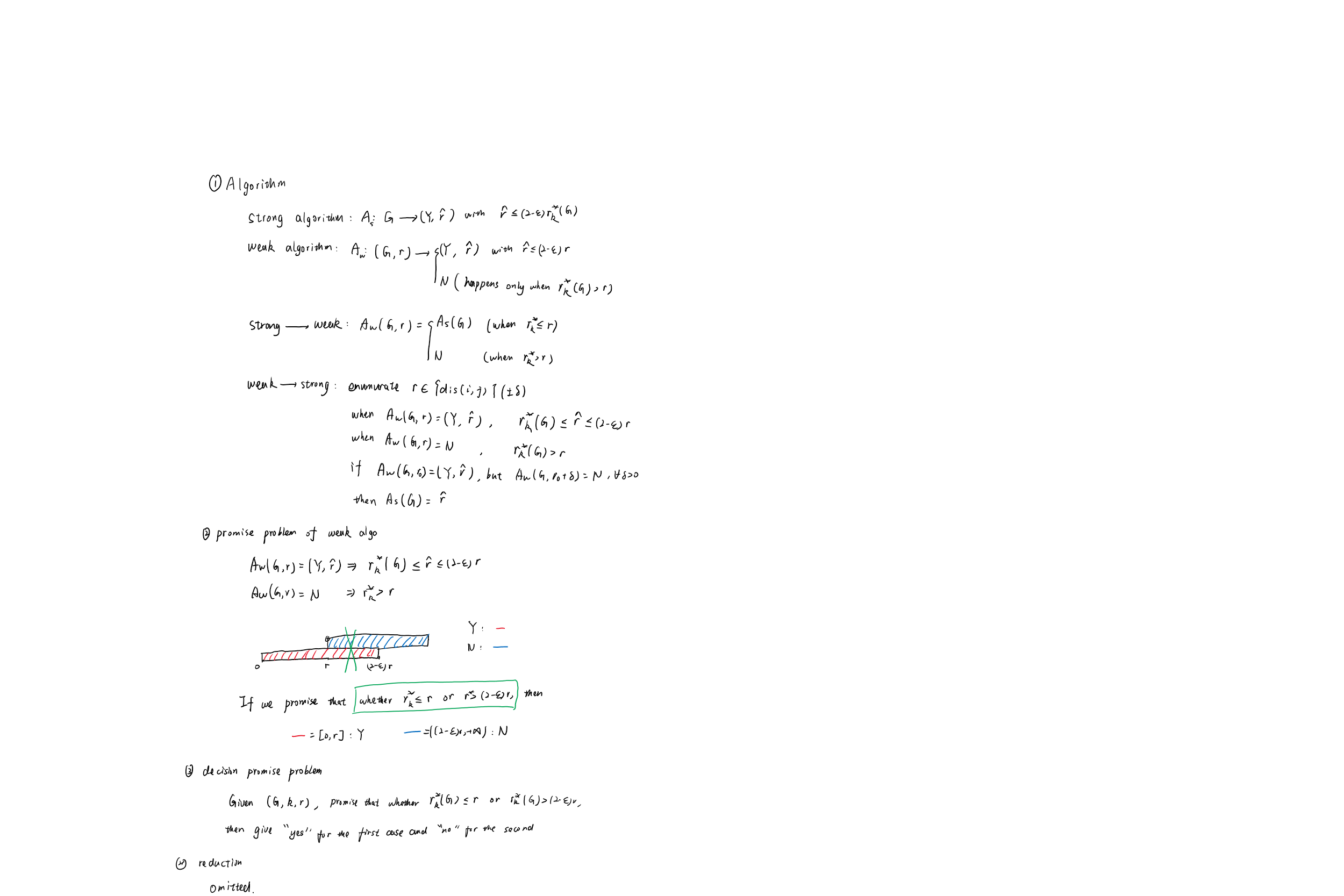# 从P和NP到k-center inapproximability

## P和NP

### 判定性问题

P问题和NP问题都是专指一类判定性问题。一般我们说一个问题是P问题或NP问题，都是说这个问题的判定性版本是P问题或NP问题。

• 最大点独立集问题：给出一张无向图 $G$，求它的最大点独立集；
• 最大点独立集问题的判定性版本：给出一张无向图 $G$ 和常数 $k$，判断这张图是否有大小不超过 $k$ 的点独立集。

• 旅行商问题：给出一张有向带边权图 $G$，找一条环路，使得经过每个点恰好一次，且边权和最小；
• 旅行商问题的判定性版本：给出一张有向带边权图 $G$ 和权值 $v$，判断是否有一条环路，使得经过每个点恰好一次，且边权和不超过 $v$

### P问题

P（Polynomial）问题即多项式问题，指存在一种算法，使得对于任意输入，都能在输入长度的多项式级别的时间内给出正确的yes和no。

### NP问题

NP（Non-deterministic Polynomial）问题即非确定性多项式问题，指存在一种算法，使得对于任意的输入，答案为yes当且仅当存在一个多项式级别的凭据，使得这个算法接收输入和凭据后会在输入长度的多项式级别的时间内给出yes。

### P=NP吗？

NP问题是P问题吗？这是一个世纪难题。遗憾的是，现有的很多NP问题都没有找到多项式级别的算法，因此目前普遍认为P $\neq$ NP，虽然仍未得到证实。

## NPC和NPH

### NP-Hard

NP-Hard是指一类问题（不一定是判定性问题），使得所有的NP问题都可以归约到这个问题。

### NP-Complete

NP-Complete问题是NP问题和NP-Hard问题的交集，即一方面问题本身是NP问题，另一方面所有的NP问题都可以归约到这个问题。上述提到的SAT问题就是一个NPC问题。

## $k$-center及其近似算法

### 定义

• $d(u,u)=0$
• $d(u,v)=d(v,u)$
• $d(u,v)\le d(u,w)+d(w,v)$

$k$-center问题被定义为：给出一张度量图 $G(V,E)$ 和正整数 $k$。一个点集 $S$ 的半径 $r(S)=\max\limits_{v\in V}\min\limits_{s\in S} d(s,v)$。你要找出一个点集 $S$， 满足 $|S|\le k$，且 $r(S)$ 最小。

### 2-近似

$k$-center问题有很多的 2-近似算法，即找到的 $r(\hat{S})\le2r^*$

• 猜测一个最优解，记为 $r_0$
• 任选一个点加入点集，并删掉所有与该点距离小于等于 $2r_0$ 的点，重复此过程直到所有的点都被删完；
• 如果最终加入点集的点数小于 $k$，则找到了一组答案为 $2r_0$ 的解；
• 否则，报告猜测的 $r_0$ 过小，无法用这个算法找到解；
• 通过不断猜测可能的 $r_0$ （可能情况只有 $n^2$ 种），最小的可行解就是一个 2-近似解。

## $k$-center inapproximability

### 强算法与弱算法

• 强算法：对于给定的 $G$$k$$A_s(G,k)$ 会给出一组解 $\hat{S}$，满足 $r(\hat{S})\le(2-\varepsilon)r^*$
• 弱算法：对于给定的 $G$$k$，以及猜测的 $r_0$，当 $r_0\ge r^*$$A_w(G,k,r_0)$ 一定会报告yes并给出一组解 $\hat{S}$，满足 $r(\hat{S})\le(2-\varepsilon)r_0$；而当 $r_0< r^ *$ 时，$A_w(G,k,r)$ 可能会返回报告no。

• 强算法 $\to$ 弱算法：直接令 $A_w(G,k,r_0)=\begin{cases}A_s(G,k)\text{, if }r(A_s(G,k))\ge(2-\varepsilon)r_0 \\ \text{no, otherwise}\end{cases}$ 即可；
• 弱算法 $\to$ 强算法：猜测所有可能的 $r_0$ （可能情况只有 $n^2$ 种），最小的可行解就是一个 $(2-\varepsilon)$-近似解，即 $A_s$ 的一组解。

### 约定性问题

• 当弱算法报告yes并给出解 $\hat{S}$ 时，一定有 $r^*\le r(\hat{S})\le(2-\varepsilon)r_0$
• 当弱算法报告no时，一定有 $r_0< r^*$

• 给出图 $G$、正整数 $k$ 以及 $r_0$保证要么 $r^ *\le r_0$，要么 $r^*>(2-\varepsilon)r_0$。判断究竟是这两种情况中的哪一种。

## Q&A

Q：这些东西有什么用？
A：帮助你更好地理解NP、NPH、NPC的关系以及$k$-center inapproximability的思考过程。
Q：这些东西有什么用？
A：帮助你更好地理解算法设计课上的内容，从而应对期中考试。
Q：这些东西有什么用？
A：……我不知道（
Q：你写的太烂了！
A：以下是原笔记：|转载请注明 [原文链接][作者] ，谢谢！

posted @ 2020-10-27 00:09  GXZlegend  阅读(403)  评论(1编辑  收藏  举报